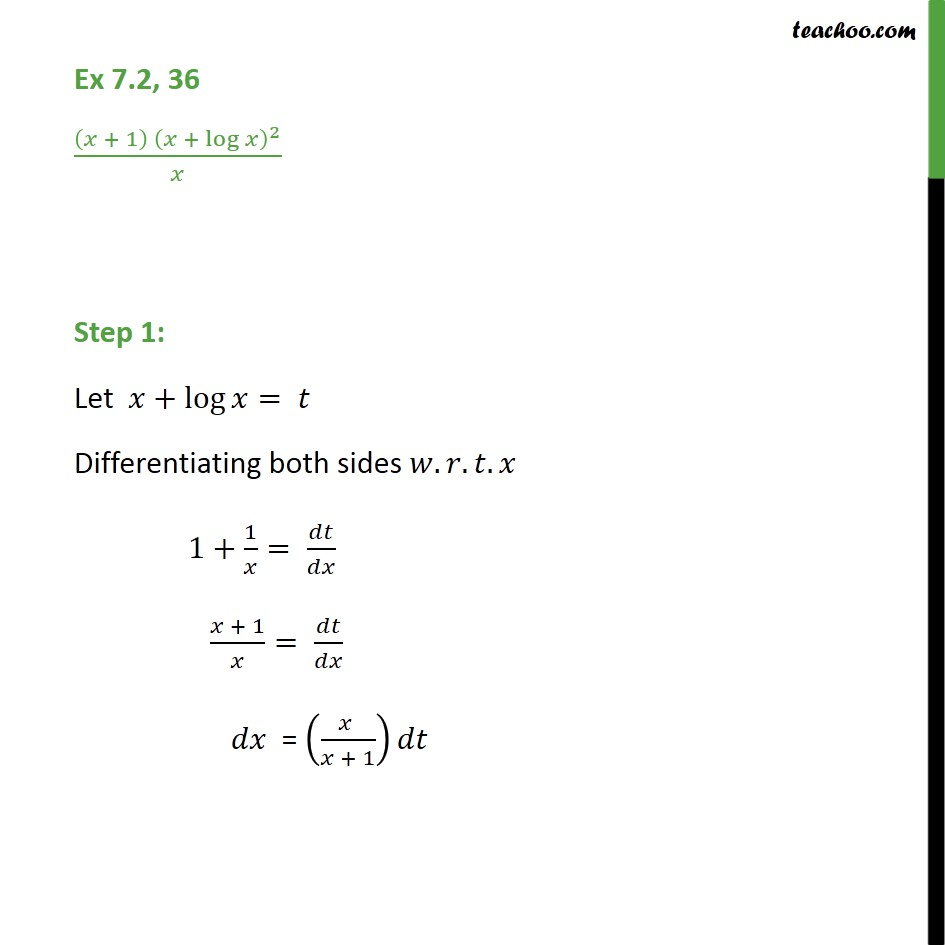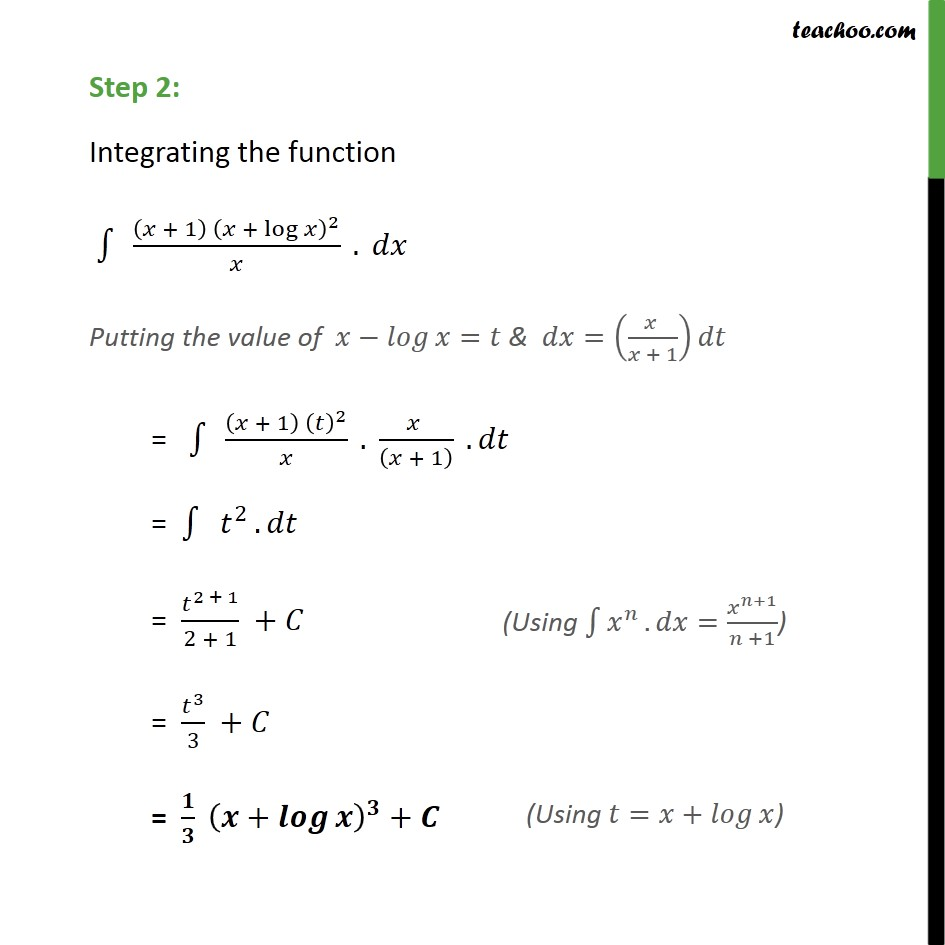1. Chapter 7 Class 12 Integrals
2. Serial order wise
3. Ex 7.2

Transcript

Ex 7.2, 36 (( + 1) ( + log )^2)/ Step 1: Let +log = Differentiating both sides . . . 1+1/ = / ( + 1)/ = / " " = (( )/( + 1)) Step 2: Integrating the function 1 " " (( + 1) ( + log )^2)/ . Putting the value of = & =(( )/( + 1)) = 1 " " (( + 1) ( )^2)/ . ( )/(( + 1) ) . = 1 " " ^2 . " " = ^(2 + 1)/(2 + 1) + = ^3/3 + = / ( + )^ +

Ex 7.2

Chapter 7 Class 12 Integrals
Serial order wise